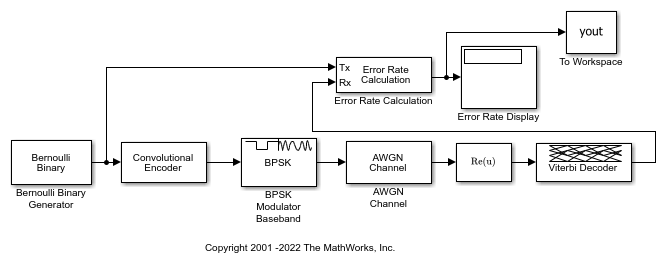# Compute SER of Convolutionally Encoded Signal

Apply convolutional encoding and BPSK modulation to a binary signal, pass the modulated signal through an AWGN channel. Compute the symbol error rate (SER) of the signal after applying BPSK demodulation and Viterbi decoding.

### Explore model

The doc_conv model generates a binary signal by using a Bernoulli Binary Generator block. The Convolutional Encoder block encodes the signal. The BPSK Modulator Baseband block modulates the signal. The AWGN Channel block adds noise to the signal. To demodulate the BPSK modulated signal with zero phase shift, simply extract the value of the real component of the complex symbol demodulation by using the Complex to Real-Imag (Simulink) block. The Viterbi Decoder block decodes the signal. The Error Rate Calculation block computes the SER.### Run simulation

```ans = 'Filtering the signal through an AWGN channel with the EsN0 set to -1 dB, the computed SER is 0.005608.' ans = 'For 53499 transmitted symbols, there were 300 symbols errors.' ```